﻿ Chapter 7: Comparing two groups using R

# Chapter 7: Comparing two groups using R

### Figure 7.1 Histogram of two groups on one graph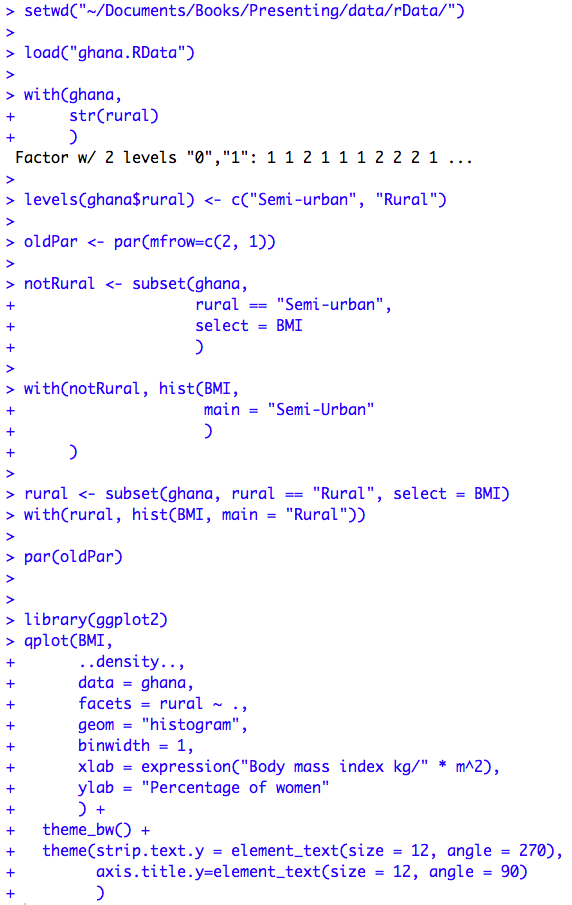### Figure 7.2 Overlapping density plots for two groups### Figure 7.3 Box and whisker plot for a continuous variable in two groups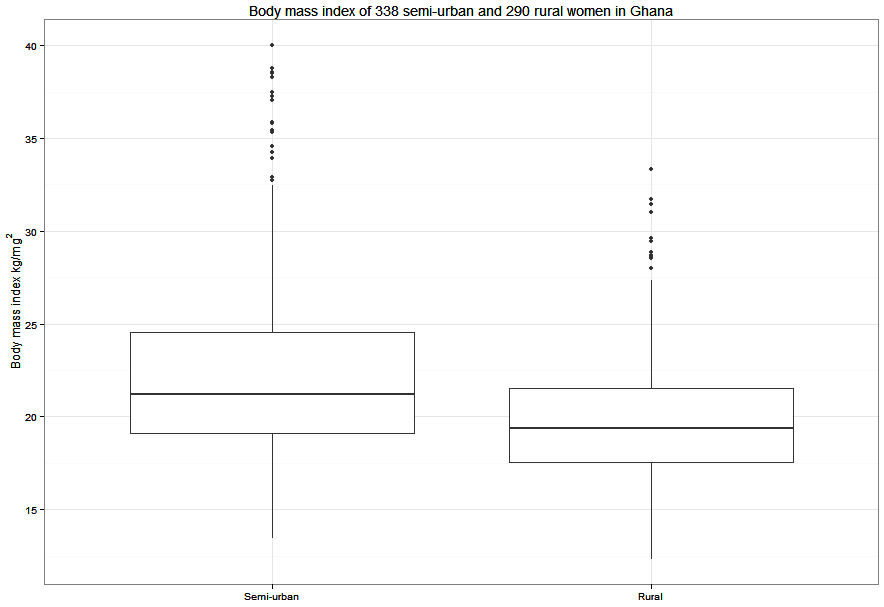### Figure 7.4 Violin plot for a continuous variable comparing two groups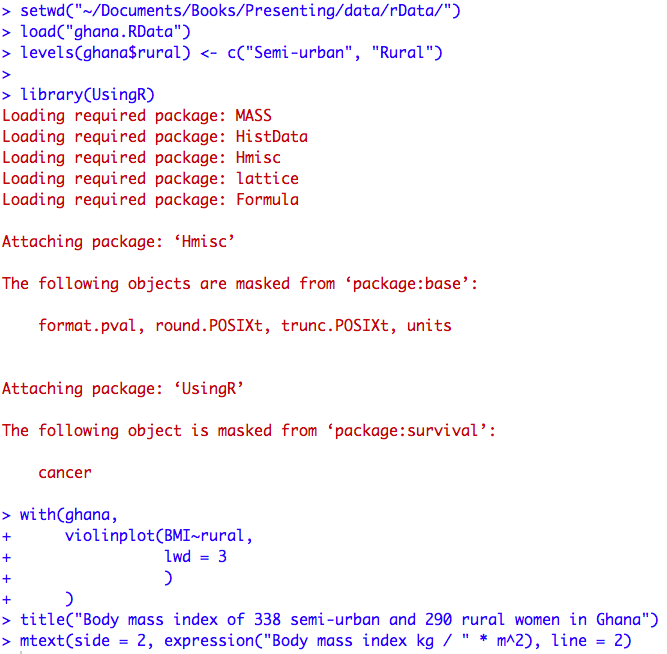### Figure 7.5 Dot plot for a continuous variable comparing two groups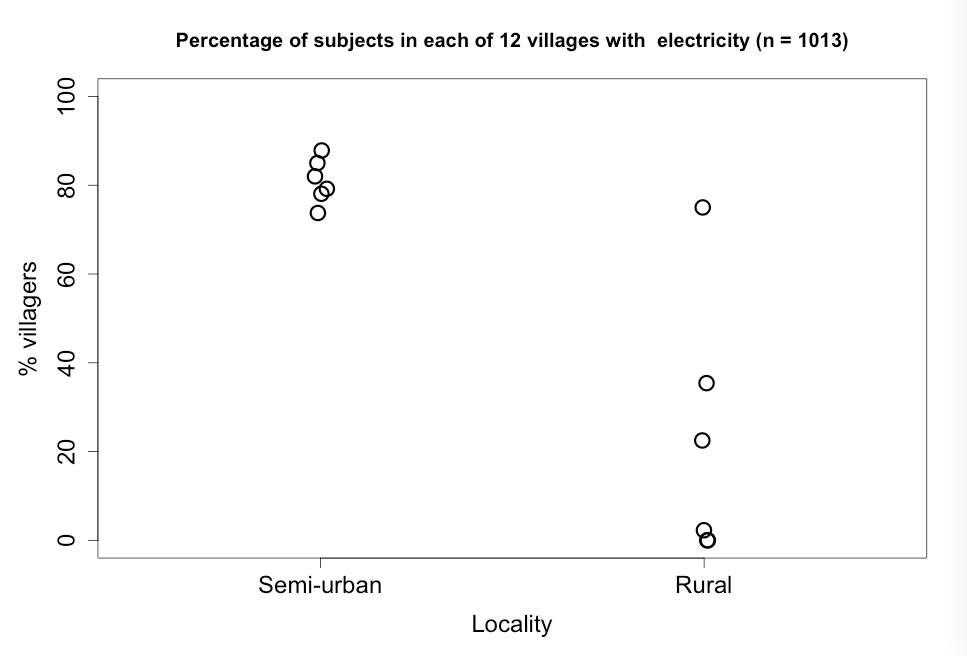### Figure 7.6 Histogram of a variable to check for normality### Figure 7.7 Output for an unpaired t-test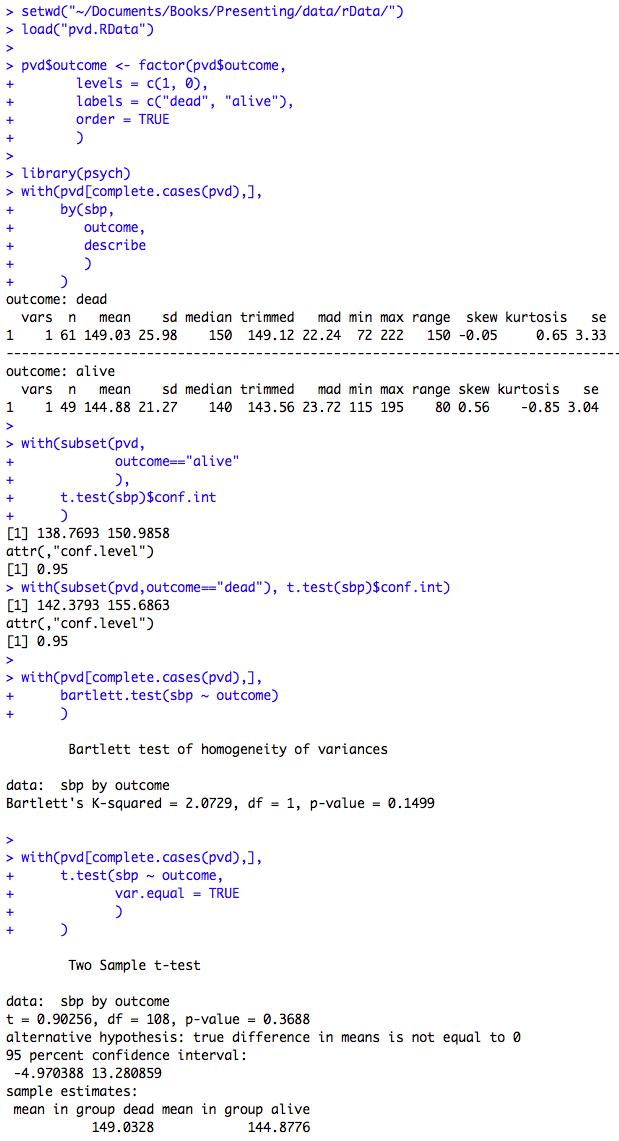### Figure 7.8 Histograms of a skewed variable before and after log transformation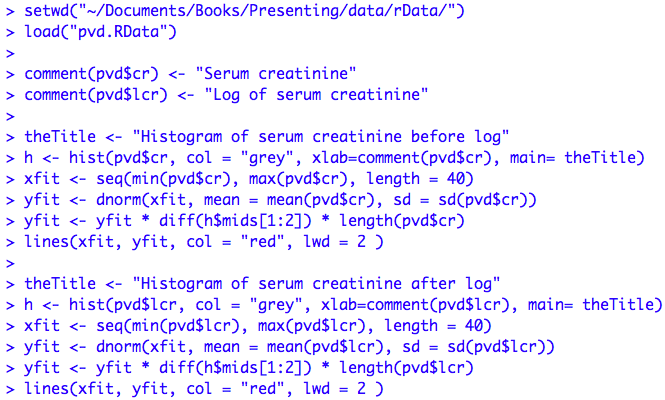### Box 7.4 Presenting the findings of a t-test on log-transformed data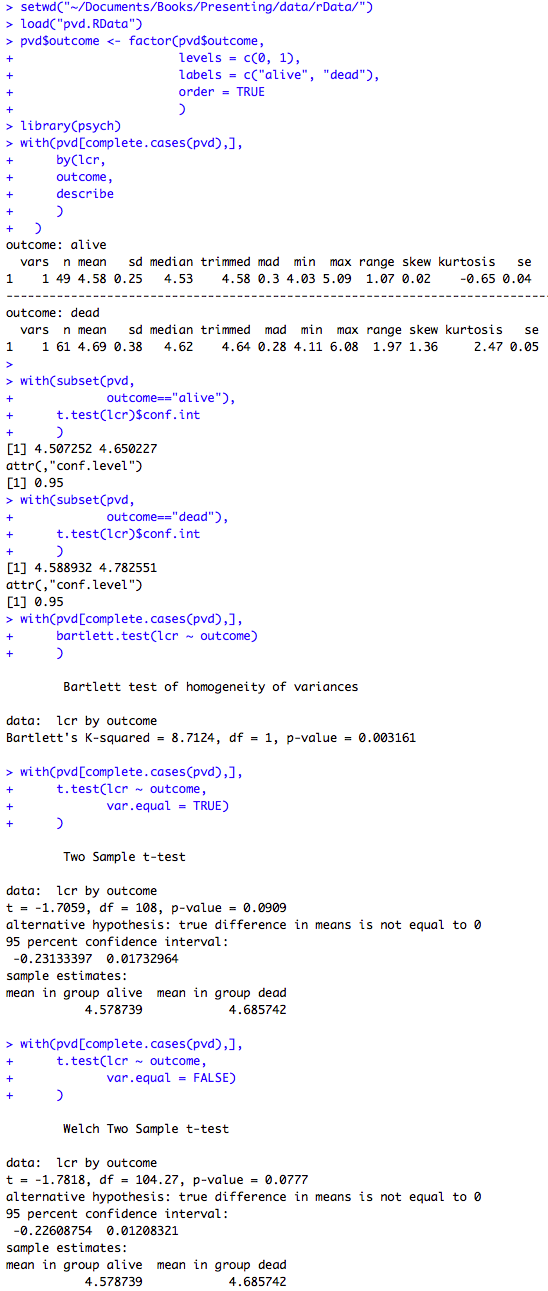### Figure 7.10 Table of data for and output from a Mann-Whitney U test### Figure 7.11 Output for chi-square test relative risk and risk difference### Figure 7.12 Output for odds ratio### Figure 7.12 Code